Ex 13.2

Chapter 13 Class 10 Surface Areas and Volumes
Serial order wise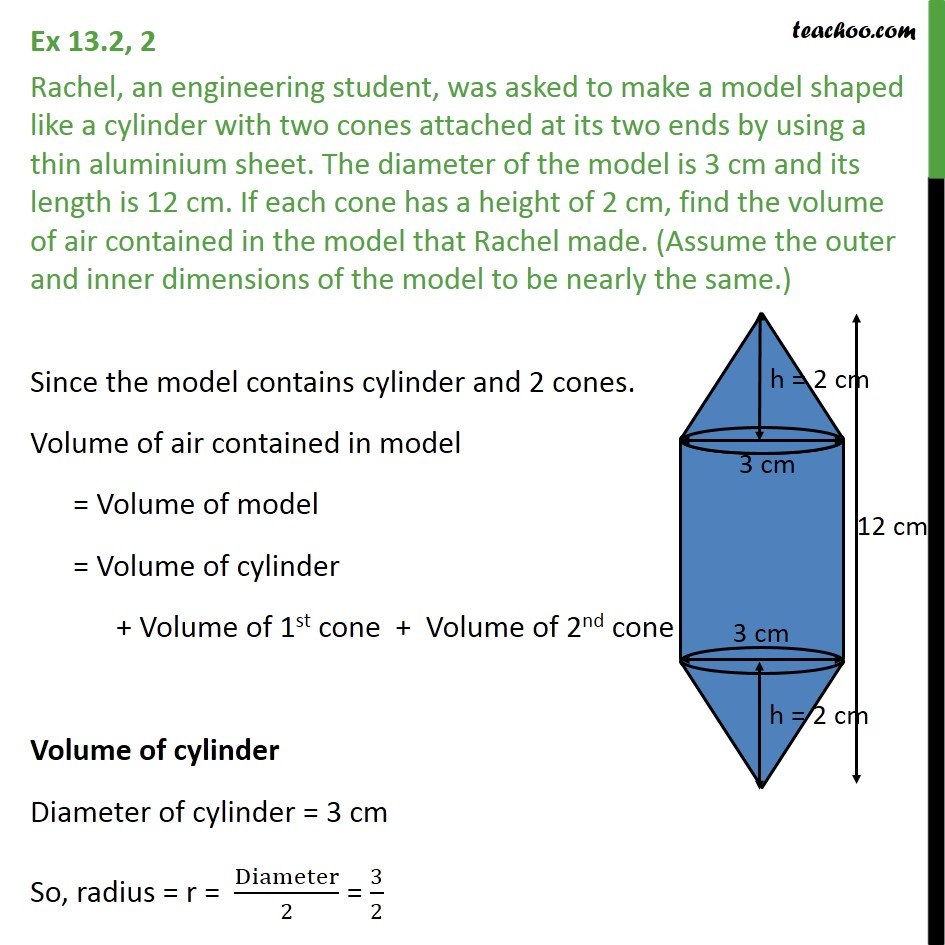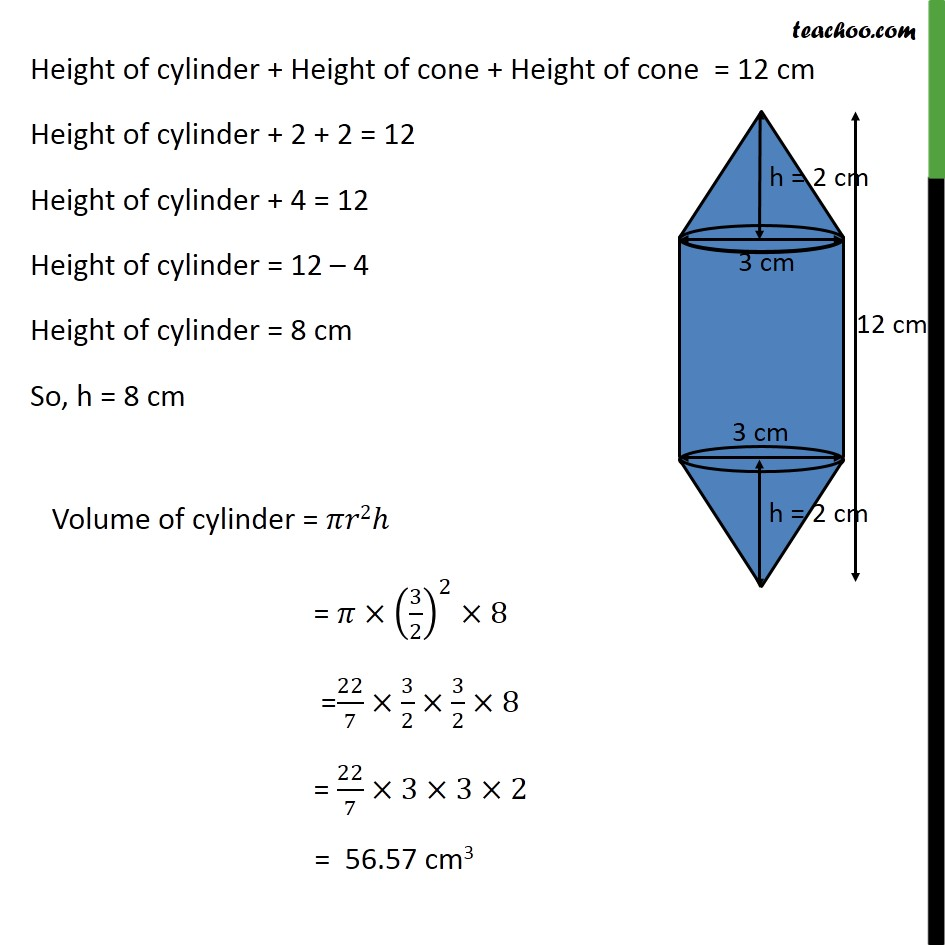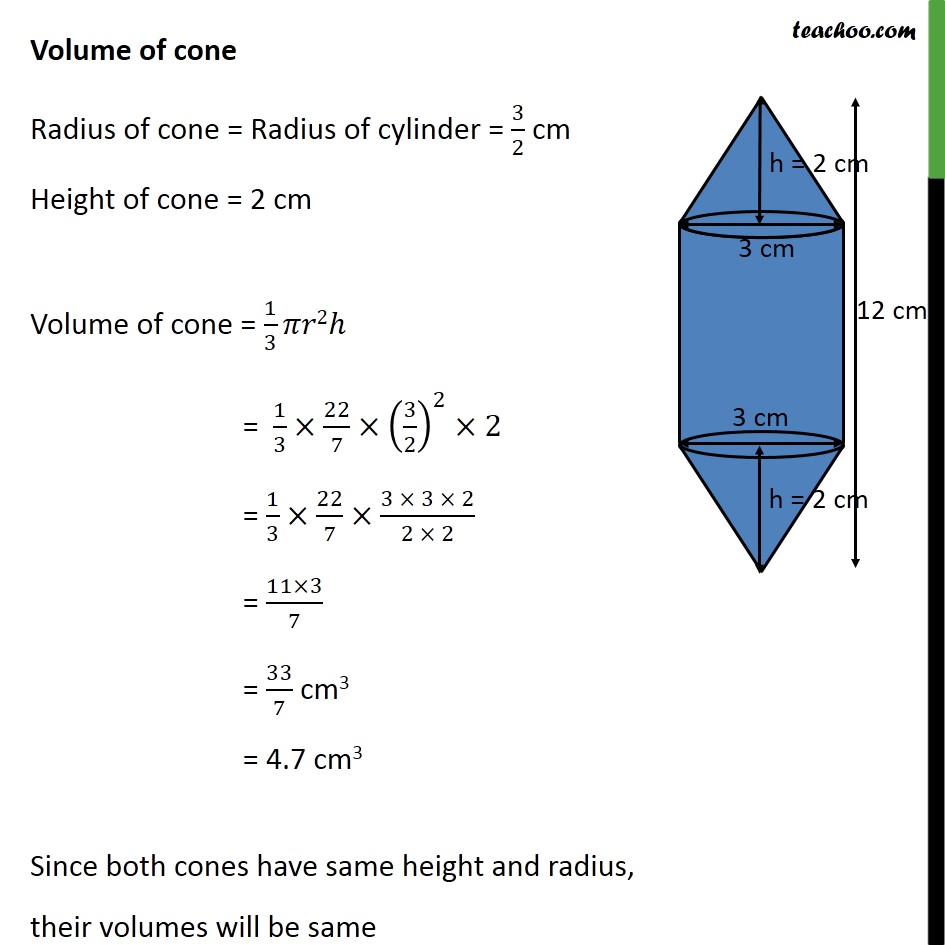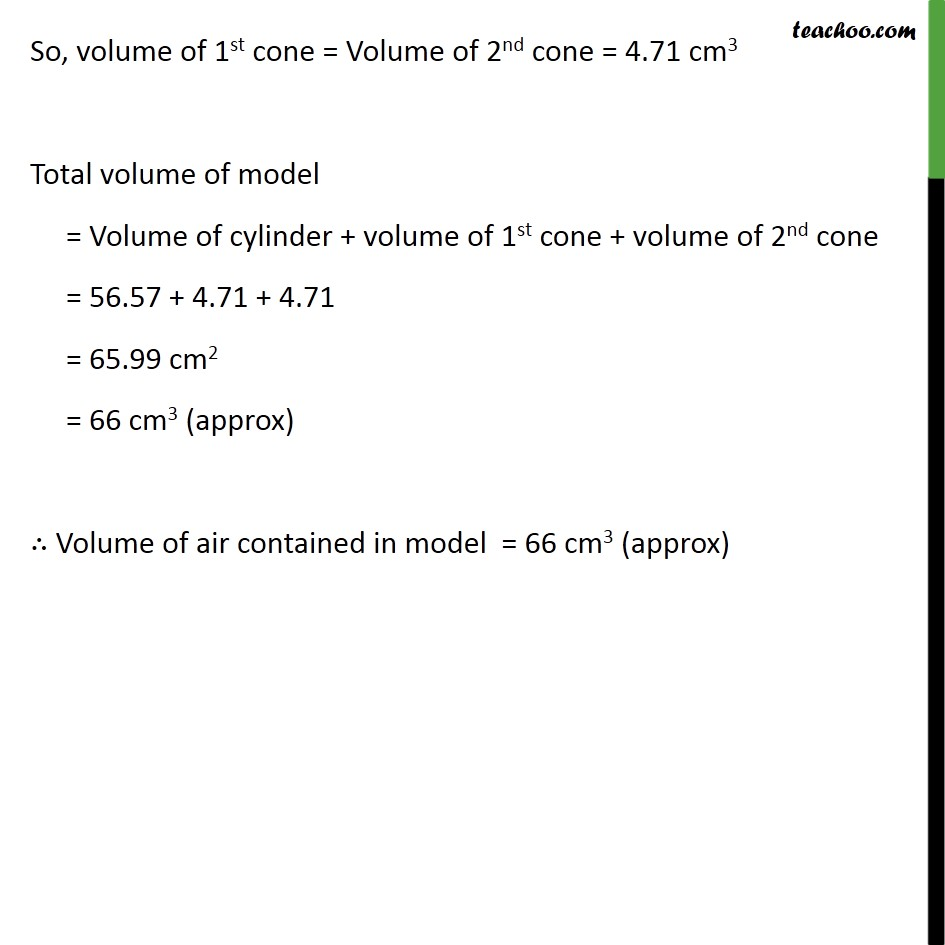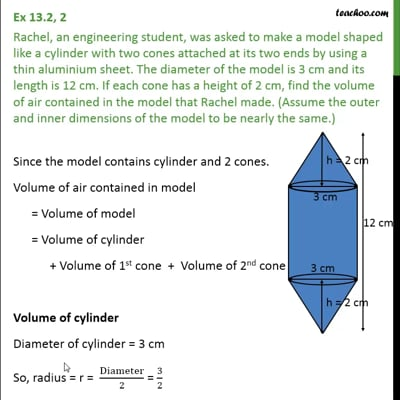This video is only available for Teachoo black users

Maths Crash Course - Live lectures + all videos + Real time Doubt solving!

### Transcript

Ex 13.2, 2 Rachel, an engineering student, was asked to make a model shaped like a cylinder with two cones attached at its two ends by using a thin aluminium sheet. The diameter of the model is 3 cm and its length is 12 cm. If each cone has a height of 2 cm, find the volume of air contained in the model that Rachel made. (Assume the outer and inner dimensions of the model to be nearly the same.) Since the model contains cylinder and 2 cones. Volume of air contained in model = Volume of model = Volume of cylinder + Volume of 1st cone + Volume of 2nd cone Volume of cylinder Diameter of cylinder = 3 cm So, radius = r = Diameter/2 = 3/2 Height of cylinder + Height of cone + Height of cone = 12 cm Height of cylinder + 2 + 2 = 12 Height of cylinder + 4 = 12 Height of cylinder = 12 – 4 Height of cylinder = 8 cm So, h = 8 cm Volume of cylinder = 𝜋𝑟2ℎ = 𝜋×(3/2)^2×8 =22/7×3/2×3/2×8 = 22/7×3×3×2 = 56.57 cm3 Volume of cone Radius of cone = Radius of cylinder = 3/2 cm Height of cone = 2 cm Volume of cone = 1/3 𝜋𝑟2ℎ = 1/3×22/7×(3/2)^2×2 = 1/3×22/7×(3 × 3 × 2)/(2 × 2) = (11×3)/7 = 33/7 cm3 = 4.7 cm3 Since both cones have same height and radius, their volumes will be same So, volume of 1st cone = Volume of 2nd cone = 4.71 cm3 Total volume of model = Volume of cylinder + volume of 1st cone + volume of 2nd cone = 56.57 + 4.71 + 4.71 = 65.99 cm2 = 66 cm3 (approx) ∴ Volume of air contained in model = 66 cm3 (approx)